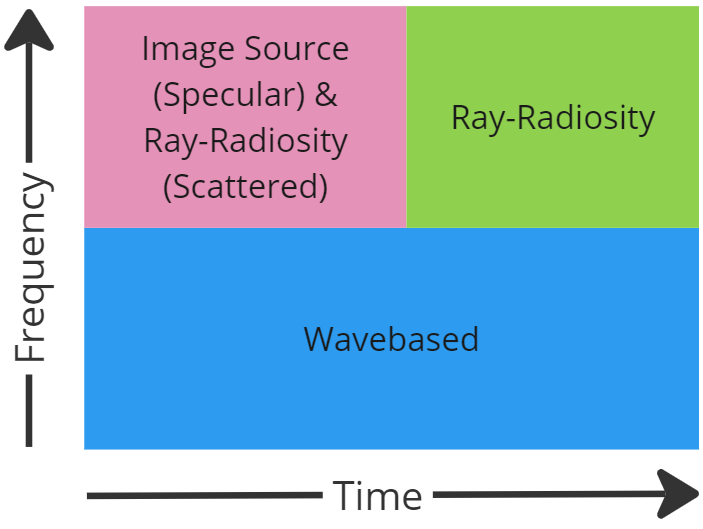# Solver concept

Covering the entire audible spectrum with the wave-based solver requires substantial compute resources and it is in most cases considered impractical, but also to some extend, unnecessary to do. Therefore Treble's state-of-the-art Geometrical Acoustics (GA) solver is used to compute the high frequency part of the acoustic response, which is later combined with the wave-based results via hybridization.

Geometrical acoustics approaches, such as the image-source and ray tracing methods date back to the 1960's [1-5] and are widely used in other commercial software, where it is considered the industry standard approach to full room acoustics modelling. In GA, the sound waves are assumed to propagate in straight lines, or as "rays". This assumption is generally fair when the wavelengths are small compared to the size of the features that the waves are interacting with. Therefore GA is well suited for simulation of high frequencies, where the wavelengths are small, and in large spaces and where the level of geometrical detail is coarse. The applied approximations and assumptions make them less accurate by nature, but on the contrary, they are generally fast and there is no added complexity or computational load in modelling high frequencies as with the wave-based methods.

Treble's GA solver uses a combined approach of an "Image Source Method(ISM)" and a "Ray-Radiosity(RR)" method. An accelerated pressure-based ISM method calculates the first specular reflection reflections and the RR method calculates the scattered energy from the first reflections along with the latter part of the energy histogram.Due to the ray assumption in GA, it is possible to include a scattering coefficient for each boundary as well as an absorption coefficient. The scattering coefficient describes how large a part of the energy in a wavefront is scattered into other directions than the specular direction, whereas the absorption coefficient denotes the amount of energy that is absorbed.

Since the ISM method is pressure based it outputs an impulse response directly. However, the output of RR method are in the form of an energy histogram that is computed pr octave band. This data is processed into an impulse response before it is combined with the ISM result to form the final impulse response.

 L. Savioja, U. P. Svensson. Overview of geometrical room acoustic modeling techniques. J. Acoust. Soc. Am., 138:708–730, 2015. https://doi.org/10.1121/1.4926438

 M. Vorländer. Auralization" Fundamentals of Acoustics, Modelling, Simulation, Algorithms and Acoustic Virtual Reality, Springer, 2008.

 Room acoustics modeling: http://interactiveacoustics.info/

 M. R. Schroeder. Novel uses of digital computers in room acoustics. J. Acoust. Soc. Am., 33:1669, 1961.

 M. R. Schroeder and K. H. Kuttruff. On frequency response curves in rooms. Comparison of experimental, theoretical, and Monte Carlo results for the average frequency spacing between maxima. J. Acoust. Soc. Am., 34:76–80, 1962.